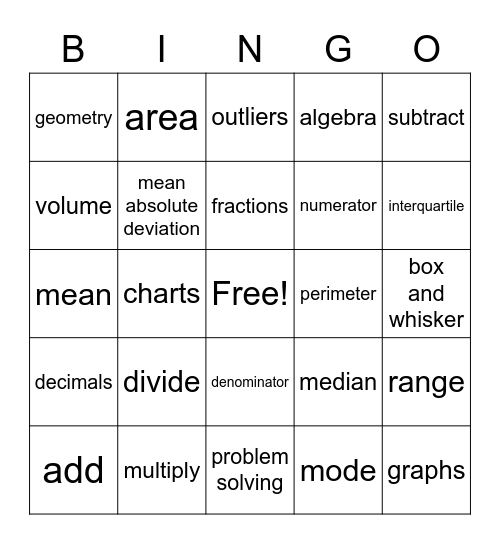# Math Lingo BingoThis bingo card has a free space and 24 words: divide, multiply, fractions, add, subtract, geometry, volume, area, perimeter, denominator, numerator, mean, median, range, mean absolute deviation, mode, box and whisker, outliers, interquartile, charts, graphs, decimals, algebra and problem solving.

## Play Online